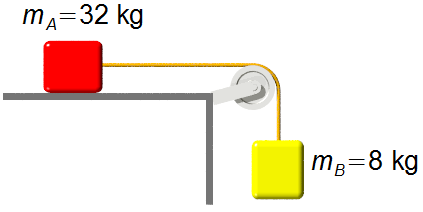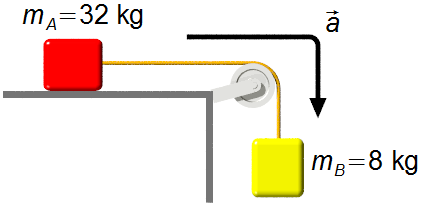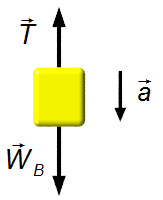Solved Problem on DynamicsPortuguêsEnglish

In the system of the figure, the body A slides on a horizontal surface without friction, dragged by body B that moves downward. Bodies A and B are attached by a rope of negligible mass parallel to the surface and passing through a frictionless pulley of negligible mass. The masses of A and B are respectively 32 kg and 8 kg. Find the acceleration of the system and the tension on the cord. Assume g = 10 m/s2.Problem data
• mass of body A:    mA = 32 kg;
• mass of body B:    mB = 8 kg;
• free-fall acceleration:    g = 10 m/s2.
Problem diagram

We choose the acceleration in the direction in which the body B moves downward. Drawing a free-body diagram for each block and using Newton's Second Law
$\bbox[#99CCFF,10px] {\vec{F}=m\vec{a}}$figure 1
Solution

Body A

Vertical direction
• $$\vec P_{\text{A}}$$: weight of body A;
• $$\vec N_{\text{A}}$$: normal force of the surface on the body.
Horizontal direction
• $$\vec T$$: tension on the cord.figure 2
In the vertical direction, the weight and the normal force cancel out, there is no vertical motion.
In the horizontal direction using Newton's Second Law we have
$T=m_{\text{A}}a \tag{I}$
Body B
• $$\vec W_{\text{B}}$$: weight of body B;
• $$\vec T$$: tension on the rope.
In the horizontal direction, there are no forces acting, in the vertical direction using Newton's Second Law we havefigure 3
$W_{\text{B}}-T=m_{\text{B}}a \tag{II}$

Expressions (I) and (II) can be written as a system of linear equations with two variables (T and a), adding the two equations we have
$\frac{ \left\{ \begin{array}{rr} \cancel{T}&=m_{\text{A}}a\\ W_{\text{B}}-\cancel{T}&=m_{\text{A}}a \end{array} \right.} {W_{\text{B}}=\left(m_{\text{A}}+m_{\text{B}}\right)a}$
$a\;=\;\frac{W_{\text{B}}}{m_{\text{A}}+m_{\text{B}}} \tag{III}$
The weight of body B is given by
$W_{\text{B}}=m_{\text{B}}g \tag{IV}$
substituting (IV) into (III) and the values given in the problem
$a=\frac{m_{\text{B}}g}{m_{\text{A}}+m_{\text{B}}}\\ a=\frac{8 \times 10}{32+8}\\ a=\;\frac{80}{40}$
$\bbox[#FFCCCC,10px] {a=2\;\text{m/s}^{2}}$
Substituting the mass of the body A and the acceleration, found above, in the first expression the tension is
$T=32 \times 2$
$\bbox[#FFCCCC,10px] {T=64\ \text{N}}$